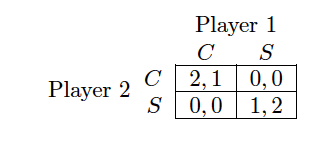# Complexity Explorer Santa Fe Institute## Game Theory I • Static Games

• What is Game Theory?
• Elements of a Game
• Normal Form Game
• Nash Equilibrium Definition
• Dominant Strategy
• Introduction and Definition
• Example
• Brief Historical Interlude
• Bayesian Games Overview
• Overfishing and Regulation
• What We've Learned and What's to Come

#### 5.3 Discussion » Quiz Solution

Question: Let p be the probability that player 2 chooses C in the game below. What is the value of p such

that player 1 is indierent between choosing C and choosing S?Answer: (d)Explanation: Player 1's utility from playing C  is. Player 1's utility from playing S  is. Setting them equal gives.